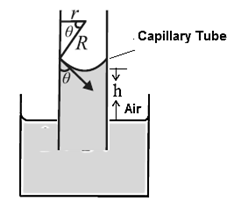# Derive an expression for capillary rise for a liquid having a concave meniscus.

more_vert

Derive an expression for capillary rise for a liquid having a concave meniscus.

more_vert

verified

Consider a capillary tube of radius r partially immersed into a wetting liquid of density p. Let the capillary rise be h and q be the angle of contact at the edge of contact of the concave meniscus and glass (Fig)..

If R is the radius of curvature of the meniscus then from the figure, r=R cosθ.Surface tension T is the tangential force per unit length acting along the contact line. It is directed into the liquid making an angle θ with the capillary wall. We ignore the small volume of the liquid in the meniscus. The gauge pressure within the liquid at a depth h, i.e. at the level of the free liquid surface open to the atmosphere, is

P— Po = ρgh ………. (1)

By Laplace’s law for a spherical membrane, this gauge pressure is

P— Po = 2T/R  …….(2)

hρg  = $$\frac{2T}{R} = \frac{2T\cosθ}{r}$$

∴ h =    $$\frac{2Tcosθ}{rρg}$$…….(3)

Thus, narrower the capillary tube, the greater is the capillary rise.

From Eq. (3),

T=  $$hrρg\over2Tcosθ$$………(4)

Equations (3) and (4) are also valid for capillary depression h of a non-wetting liquid. In this case, the meniscus is convex and θ is obtuse. Then, cosθ is negative but so is h, indicating a fall or depression of the liquid in the capillary. T is positive in both cases.

[For capillary rise, Eq. (3) is also called the ascent formula. ]

## Related questions